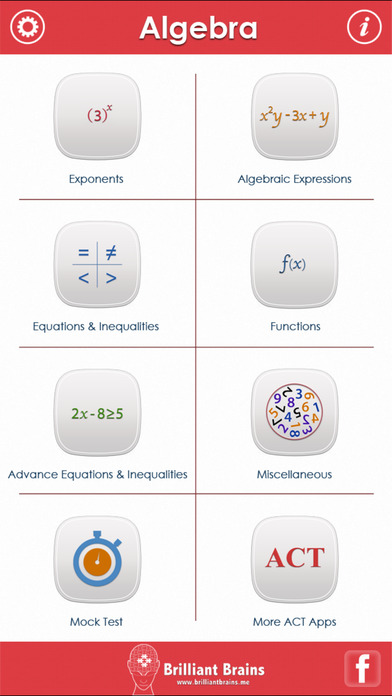ACT Math : Algebra Lite

iOS UniversalEducation

Free

490 questions and 49 revision notes covering all ACT Algebra syllabus. This is a high quality content written by an experienced Mathematician.

Other features include:

** MOCK TEST
Mixed questions from all topics.

** INSTANT FEEDBACK
Know instantly if your answer was right or wrong.

** REVIEW WITH EXPLANATION
Review each question at the end of the test. Know the right answer with detailed explanation for each question.

** PROGRESS METER
With our unique progress tracking feature including pie charts and bar graphs showing your progress, you know you are ready to take on the real test at the board when your progress meter says 100%.

** REVISE BY TOPIC

1. Exponents

Exponents of 10
Scientific Notation
Square Roots
Squares
Evaluating Exponents
Dividing Exponents
Multiplying Exponents
Exponent of a Exponent

2. Algebric Expressions

Addition and Subtraction of Algebraic Expressions
Multiplication of Algebraic Expressions
Algebraic Fractions
Expanding Simplifying and Factoring
Binomial Expansion
Evaluating Expressions 1
Evaluating Expressions 2
Manipulation and Evaluation of Expressions
Translation into Algebraic Expressions
Substitution Problems
Difference of Two Squares
Factoring Quadratics with Unit Coefficient

3. Equations & Inequalities

Linear Equations 1
Linear Equations 2
Linear Equations 3
Inequalities
Inequalities on Number Lines
Solving Inequalities
Solving for One Variable in Terms of Another
Setting up Equations
Solving Equations with Radical Expressions
Working with 'unsolvable' equations
Equations Involving Absolute Values

4. Functions

Functions as Models
Compound Functions and Translations
Logarithms

5. Advance Equations & Inequalities

Systems of Equations (Linear)
Setting up Systems of Equations
Solving Quadratics by Factoring
Using the Quadratic Formula
Rational Equations and Inequalities

6. Miscellaneous

Introduction to Sequences
Arithmetic Sequences
Geometric Sequences
Surds
Complex Numbers
Matrices

Disclaimer:
ACT is a registered trademark of ACT Inc. ACT Inc neither sponsors nor endorses this product or any of its content.
There may be a possibility of some bugs, inaccuracies or typographical errors for which the owner cannot be held liable.

What's New

Updated data and code to work with latest iOS version.

•••••